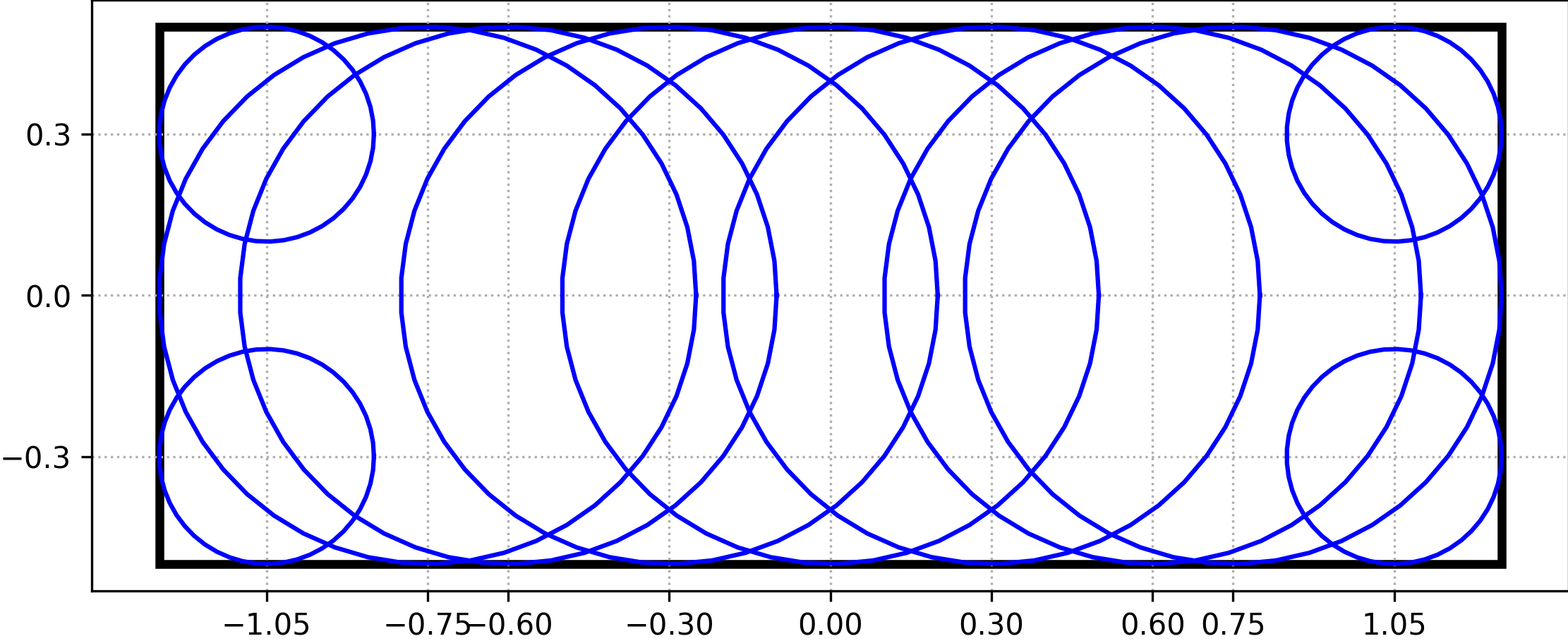# microstructpy.geometry.Rectangle¶

class microstructpy.geometry.Rectangle(**kwargs)[source]

This class contains a generic, 2D rectangle. The position and dimensions of the box can be specified using any of the parameters below.

Without parameters, this returns a unit square centered on the origin.

Parameters
• length (float) – (optional) Length of the rectangle.

• width (float) – (optional) Width of the rectangle. (optional)

• side_lengths (list) – (optional) Side lengths. Defaults to (1, 1).

• center (list) – (optional) Center of rectangle. Defaults to (0, 0).

• corner (list) – (optional) Bottom-left corner.

• bounds (list) – (optional) Bounds of rectangle. Expected to be in the format [(xmin, xmax), (ymin, ymax)].

• limits – Alias for bounds.

• angle (float) – (optional) The rotation angle, in degrees.

• angle_deg (float) – (optional) The rotation angle, in degrees.

• matrix (numpy.ndarray) – (optional) The 2x2 rotation matrix.

approximate(x1=None)[source]

Approximate rectangle with a set of circles.

This method approximates a rectangle with a set of circles. These circles are spaced uniformly along the long axis of the rectangle with distance x1 between them.

Example

For a rectangle with length=2.5, width=1, and x1=0.3, the approximation would look like Fig. 29.Fig. 29 Circular approximation of rectangle.

Parameters

x1 (float or None) – (optional) Spacing between the circles. If not specified, the spacing is 0.25x the length of the shortest side.

Returns

An Nx3 array, where each row is a circle and the columns are x, y, and r.

Return type

numpy.ndarray

classmethod area_expectation(**kwargs)[source]

Expected area of rectangle

This method computes the expected area of a rectangle. There are two main ways to define the size of a rectangle: by the length and width and by the bounds. If the input rectangle is defined by length and width, the expected area is:

$\mathbb{E}[A] = \mathbb{E}[L W] = \mu_L \mu_W$

For the case where it is defined by upper and lower bounds:

$\mathbb{E}[A] = \mathbb{E}[(X_U - X_L) (Y_U - Y_L)]$
$\mathbb{E}[A] = \mu_{X_U}\mu_{Y_U} - \mu_{X_U} \mu_{Y_L} - \mu_{X_L}\mu_{Y_U} + \mu_{X_L}\mu_{Y_L}$

Example

>>> import scipy.stats
>>> import microstructpy as msp
>>> L = scipy.stats.uniform(loc=1, scale=2)
>>> W = scipy.stats.norm(loc=3.2, scale=5.1)
>>> L.mean() * W.mean()
6.4
>>> msp.geometry.Rectangle.area_expectation(length=L, width=W)
6.4

Parameters

**kwargs – Keyword arguments, same as Rectangle but the inputs can be from the scipy.stats module.

Returns

Expected/average area of rectangle.

Return type

float

best_fit(points)[source]

Find rectangle of best fit for points

Parameters

points (list) – List of points to fit.

Returns

an instance of the class that best fits the points.

Return type

Rectangle

plot(**kwargs)[source]

Plot the rectangle.

This function adds a matplotlib.patches.Rectangle patch to the current axes. The keyword arguments are passed through to the patch.

Parameters

**kwargs (dict) – Keyword arguments for the patch.

within(points)

Test if points are within n-box.

This function tests whether a point or set of points are within the n-box. For the set of points, a list of booleans is returned to indicate which points are within the n-box.

Parameters

points (list or numpy.ndarray) – Point or list of points.

Returns

Flags set to True for points in geometry.

Return type
property angle

Rotation angle of rectangle - degrees

Type

float

property angle_deg

Rotation angle of rectangle - degrees

Type

float

property angle_rad

Rotation angle of rectangle - radians

Type

float

property area

Area of rectangle

Type

float

property bounds

(lower, upper) bounds of the box

Type

list

property corner

bottom-left corner

Type

list

property length

Length of rectangle, side length along 1st axis

Type

float

property limits

(lower, upper) bounds of the box

Type

list

property n_dim

Number of dimensions, 2

Type

int

property n_vol

area, volume of n-box

Type

float

property sample_limits

(lower, upper) bounds of the sampling region of the box

Type

list

property width

Width of rectangle, side length along 2nd axis

Type

float